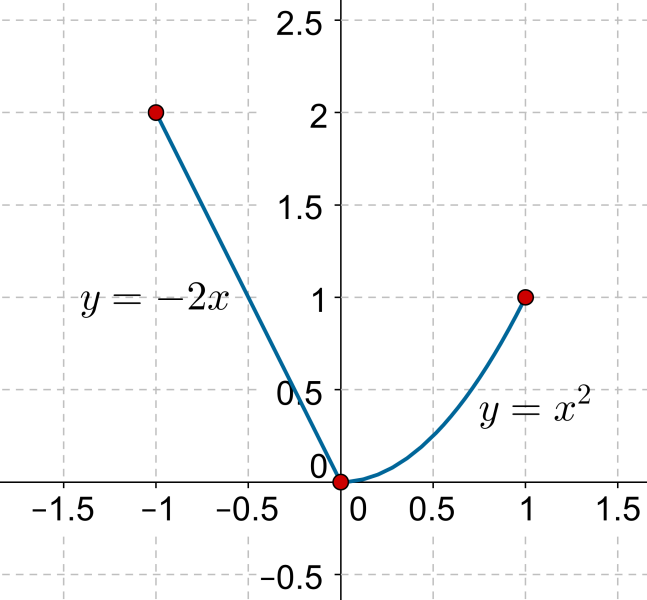# Piecewise-Defined Functions

Go back to  'Functions'

Consider the following function definition:

$f\left( x \right) = \left\{ \begin{array}{l} - 2x, & - 1 \le x < 0\\{x^2}, & 0 \le x < 1\end{array} \right.$

This is an example of a function defined piecewise, which means that the function definition is different on different parts of its domain. For the function above, the domain is $$\left[ { - 1,1} \right]$$, but the function definition on $$\left[ { - 1,0} \right)$$is different from the function definition on $$\left[{0,1} \right]$$.

The following figure shows the graph of this function:The range of this function is $$\left[ {0,2} \right]$$.

Example 1: Consider the following function:

f\left( x \right) = \left\{ \begin{align}&\;\;\;\; 1, & x \in \mathbb{Q}\\ &- 1, & x \notin \mathbb{Q} \end{align} \right.

Find the value of $$f\left( 1 \right)$$, $$f\left( {\sqrt 2 } \right)$$, and $$f\left( \pi \right)$$.

Solution: The function definition says that if the input is a rational number, then the output is 1, else the output is $$- 1$$. Thus,

$\begin{array}{l}f\left( 1 \right) = 1\\f\left( {\sqrt 2 } \right) = f\left(\pi \right) = - 1\end{array}$

Functions
Functions
grade 10 | Questions Set 2
Functions
Functions
grade 10 | Questions Set 1